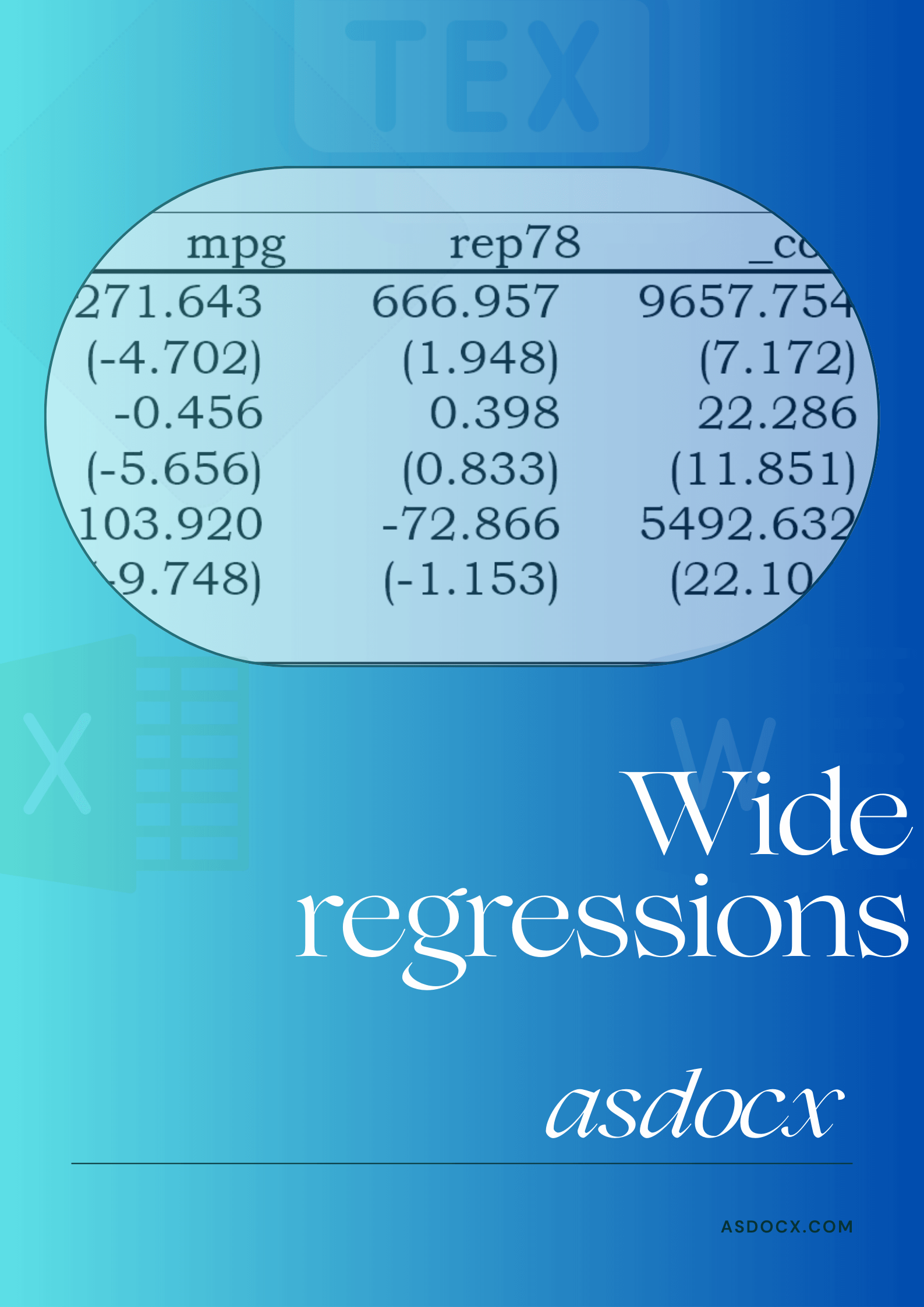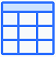### Create detailed, nested, or wide regression tables in Stata with asdocx.

asdocx tables are nicely formatted, highly customizable, can be exported to Word, Excel, LaTeX, or HTML formats.

asdocx: Your essential tool for diverse regression tables in research, spanning detailed, nested, wide, to reg1 template.

#Wide Regression tables

Option wide can be used with regression commands for making wide regression tables. Such tables can have functional usefulness to accommodate many regressions and can look great. Wide tables can be used in many circumstances; however, I mention one of them below:

Portfolios / industry / country type regressions. Let’s say that we have 20 portfolios and want to regress each portfolio returns on the same risk factors, that might include  MKTrf , SMB , and  HML . The only variable that change in each regression is the portfolio returns. These 20 regressions can be aesthetically shown using wide regression table where each regression is reported in a row. All regressions are stacked over one another.

##Customization Options for Wide Regression Tables

The following options are available with the option wide. These options are further explained with the help of examples in the following section.

###1. No t – values or p – values

Example: Default output style for wide regressions


sysuse auto
asdocx reg price mpg rep78, replace wide
ㅤ
* Add another regression where the dependent variable is trunk
asdocx reg trunk mpg rep78, wide
ㅤ
* Add third regression where the dependent variable is weight
asdocx reg weight mpg rep78, wide 
\text{ Table: asdocx wide table}
Vars mpg rep78 Const. R^2
price -271.643 666.957 9657.754 0.251
trunk -0.456 0.398 22.286 0.343
weight -103.920 -72.866 5492.632 0.656
\text{ \footnotesize{ Notes: Table was created by asdocx using option wide }} :

###2. Reporting se / t-values below coefficients


sysuse auto
asdocx reg price mpg rep78, wide replace t(below)
ㅤ
* Add another regression where the dependent variable is trunk
asdocx reg trunk mpg rep78, wide t(below)
ㅤ
* Add third regression where the dependent variable is weight
asdocx reg weight mpg rep78, wide t(below) 

Note: we can report standard errors (se) instead of t-values by replacing t(below) with se(below) in the above examples.

\text{Table: Wide table with t-values below coefficients}
Vars mpg rep78 Const. R^2
price -271.643 666.957 9657.754 0.251
\text{ t-value } (-4.702) (1.948) (7.172)
trunk -0.456 0.398 22.286 0.343
\text{ t-value } (-5.656) (0.833) (11.851)
weight -103.920 -72.866 5492.632 0.656
\text{ t-value} (-9.748) (-1.153) (22.104)
\text{\footnotesize{ Notes: Report t-values using option t(below)}} :

###3. Reporting se / t – values side-ways


sysuse auto
asdocx reg price mpg rep78, wide replace t(side)
ㅤ
* Add another regression where the dependent variable is trunk
asdocx reg trunk mpg rep78, wide t(side)
ㅤ
* Add third regression where the dependent variable is weight
asdocx reg weight mpg rep78, wide t(side) 

Note: we can report standard errors (se) instead of t-values by replacing t(side) with se(side) in the above examples.

\text{Table: Reporting t-values sideways}
Vars mpg rep78 _cons t(mpg) t(rep78) t(Const.) R^{2}
price -271.643 666.957 9657.754 (-4.702) (1.948) (7.172) 0.251
trunk -0.456 0.398 22.286 (-5.656) (0.833) (11.851) 0.343
weight -103.920 -72.866 5492.632 (-9.748) (-1.153) (22.104) 0.656
\text{\footnotesize{Notes: Report t-values sideways using t(side)}}
3.1 Option bracket: The default is to use parenthesis around standard errors / t-values. However, we can use option bracket to use square bracket around these values.
3.2 Option notse The default is to report t-values or se text in each alternate row when option t(below) or se(below) is used. This text can be suppressed with option notse.

###4. Report stars for significance

Option stars adds asterisks with regression coefficients such that *** are added for 1%, ** for 5%, and * for 10% level of significance.


sysuse auto
asdocx reg price mpg rep78, wide replace stars
ㅤ
asdocx reg trunk mpg rep78, wide stars
asdocx reg turn mpg rep78, wide stars
asdocx reg weight mpg rep78, wide stars
asdocx reg headroom mpg rep78, wide stars
\text{Table: Wide regression table with significance stars}
\text{Vars} \text{mpg} \text{rep78} \text{Const.} R^{2}
\text{price} -271.643*** 666.957* 9657.754*** 0.251
\text{trunk} -0.456*** 0.398 22.286*** 0.343
\text{turn} -0.484*** -1.072*** 53.752*** 0.589
\text{weigh}t -103.920*** -72.866 5492.632*** 0.656
\text{\footnotesize{Notes: In the wide regression tables, use option }} \textit{stars} \text{ \footnotesize{ for reporting significance}}

###5. Report additional regression statistics

Option stat() can be used to report additional regression statistics that are stored in macro help e(). For example, to report RMSE and RSS of a regression, we shall add option stat(rmse, rss). Some of the most commonly used e() statistics of regression models are as follows. Please note that any other statistics from macro e() can be reported in addition to the following.

Option Purpose
N Number of observations. Reported by asdocx as default
r2 R-squared. Reported by asdocx as default
r2_a Adjusted r-squared
F F-statistics
rmse RMSE
rss Residual sum of squares
ll Log-likelihood
chi2 Chi-square value

Example : Report N, adjusted R2, and RMSE


* Report N, adjusted R2, and RMSE
asdocx reg price mpg rep78, wide stat(N rmse r2_a) replace

###6. Suppress R2

The r-squared ( R2 ) is reported by default in wide regressions. However, it can be suppressed by option nor2. For example:

* Do not report R2
asdocx reg price mpg rep78, wide nor2 replace

###7. Add custom row text

Option add(text) can be used to specify the text that appears in the first cell of each row. By default, asdocx reports name of the dependent variable in the first cell of each row. See the following examples where the dependent variables are price and trunk in the two regressions, respectively. We shall report the text Regression 1 and Regression 2 instead.

sysuse auto
asdocx reg price mpg rep78, wide replace add(Regression 1)
ㅤ
* Add another regression where dependent variable is trunk
asdocx reg trunk mpg rep78, wide add(Regression 2) 

###8. Add custom column text

Option cnames(text) can be used to specify the text that appears in the header column. By default, asdocx reports name of the independent variable in the header column. If we were to use a different text, we shall use option cnames(text1 text2 ...). For example

 asdocx reg price, wide cnames(Abnormal_ret) 

###9. Start a new table within the same file

Using option newtable, we can start a new table within the existing file. So let us write two regressions to Table 1 and two more regressions to Table 2 in the same file.

sysuse auto
asdocx reg price mpg rep78, wide replace
asdocx reg trunk mpg rep78, wide

Start a new table within the same file, this time we are not using option replace. Please note the first line that works as a title for the new table. The option fs(14) actually makes the font size bigger than the normal text, that has a default value of 10. Please also note the RTF control word \par at end of the sentence, that marks a new paragraph in the document and ends the previously started table.

 asdocx, text(My New Table \par) fs(14)
ㅤ
* Start a new table with option newtable
asdocx reg length mpg rep78, wide newtable
asdocx reg trunk mpg rep78, wide 

###10. End a table

Using option end, asdocx will add the following text after the last row of the table.
a. If option t() is used, then the text “t-statistics are parentheses” will be added.
b. If option se() is used, then the text “Standard errors are in parentheses will be added.”
c. If option stars  is used, then the text “*** p<0.01, ** p<0.05, * p<0.1 will be added.”
If option end is not used, asdocx will still report complete table, however, the above text will not be added to the end of the table.

###11. Using parenthesis as a text with option btp

Since asdocx uses parenthesis “()” as parsing characters, it can be tricky to use them as simple text. Option btp allows converting square brackets to parentheses when they are written to the Word file. For example, if we use option add(text) and use parenthesis inside this option, this will confuse the parsing process. Therefore, we shall play a trick to first use square brackets in the text and then convert them back to parentheses. Therefore, if we write the text


* Replace parentheses with brackets
asdocx reg mpg price trunk, wide add(Millege[mpg]) replace btp

##Regressions over groups using  by() option

Regression tables in a wide format are particularly suitable for regressions across distinct groups. Consider a dataset encompassing variables such as years, industries, countries, or similar categorical divisions. When you run regressions for these groups, the only thing that changes is the group you’re focusing on. All the other variables in the regression model stay the same. Such regressions can be efficiently represented using wide regression tables.

We’ll illustrate with a dataset sourced from the web, named  grunfeld . This dataset comprises variables representing companies, years, and three additional attributes:  invest mvalue kstock . To demonstrate the utility of wide regressions with this dataset, let’s assume we have the following cross-sectional regression model, which we aim to run annually:

invest = \alpha + \beta_1 invest + \beta_2 mvalue+ \beta_3 kstock+\epsilon \;\;\;........\;\;\; (Eq.1)
 * Load data from the web
webuse grunfeld, clear
ㅤ
* Make wide regression table for regressions over years
bys year: asdocx reg invest mvalue kstock, wide replace`
\text{Table: Wide regressions table for regressions over groups}
Year mvalue kstock Const. R^{2}
1935 0.102 -0.002 0.356 0.865
1936 0.084 -0.054 15.219 0.696
1937 0.077 0.218 -3.386 0.664
1938 0.068 0.269 -17.582 0.706
1939 0.066 0.199 -21.154 0.827
1940 0.095 0.202 -27.047 0.839
1941 0.115 0.177 -16.519 0.856
1942 0.143 0.071 -17.618 0.857
1943 0.119 0.105 -22.764 0.842
1944 0.118 0.072 -15.828 0.876
1945 0.108 0.050 -10.520 0.907
1946 0.138 0.005 -5.991 0.895
1947 0.164 -0.004 -3.732 0.891
1948 0.179 -0.043 8.539 0.789
1949 0.162 -0.037 5.178 0.863
1950 0.176 -0.022 -12.175 0.858
1951 0.183 -0.112 26.138 0.874
1952 0.199 -0.067 7.293 0.846
1953 0.183 0.099 -50.153 0.889
1954 0.135 0.331 -133.393 0.898
\text{\footnotesize{Notes: Wide regressions over groups}}